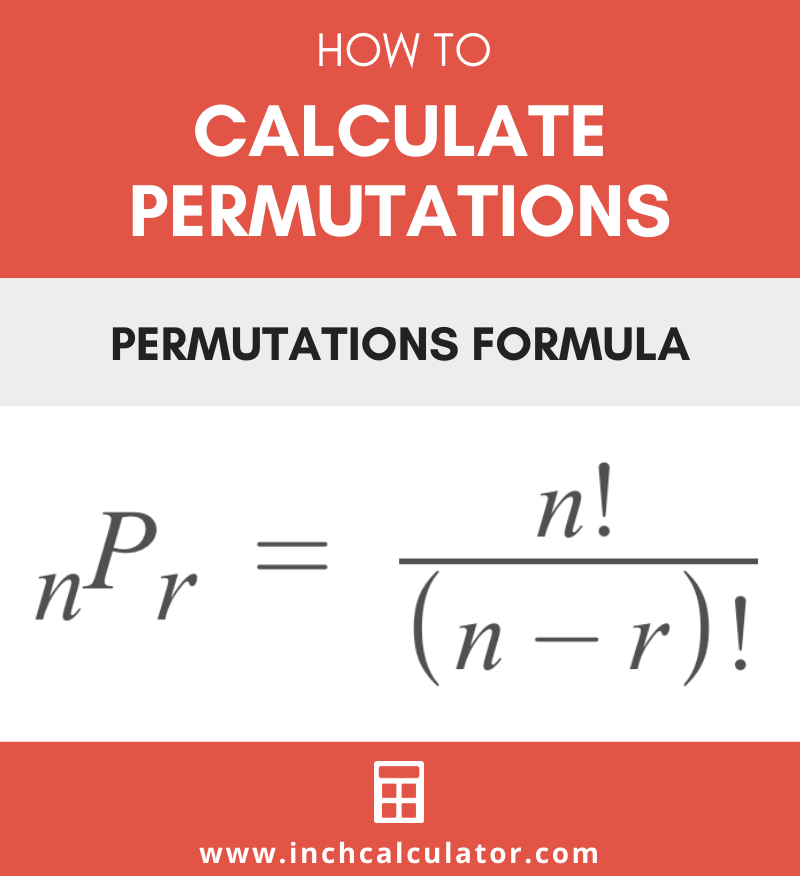# Permutation Calculator

Enter the total number of objects and the sample size to calculate the number of possible permutations.

## Result:

### Number of Combinations C(n,r)

Learn how we calculated this below

## How to Calculate Permutations

A permutation is a group of items from a larger set in a specific, linear order. The number of possible permutations for items in a set is often represented as nPr or k-permutations of n.

A permutation is basically one possible way to represent a sample of items in a particular order from a large set. There is a formula to calculate the number of possible permutations of items in a set.

Once you know the number of permutations of a set, you can calculate the probability of each one of them occurring.

### Permutation Formula

The following formula defines the number of possible permutations of r items in a collection of n total items.

P(n,r) = n! / (n – r)!

Thus the number of permutations of r items in a set of n items is equal to n factorial divided by n minus r factorial.

## Permutations with Repetitions

So far, the formulas to calculate permutations have not allowed any repetition in the sample, and the assumption has been that each element is unique. But in some cases, you may want to allow for the repetition of duplicate values.

For example, let’s say you have the letters “FOORT”. If you want to find the number of three-letter words you can make using these five letters, you might consider that the duplicate “O”s do not form different words.

For instance, “ROT” and “ROT” using the different “O”s are the same word, so they would not be counted as separate permutations in this example. The permutations formula above will calculate the number of permutations without repetitions.

However, what if you want to consider that the words “ROT” and “ROT” using the different “O”s are different variations? The formula to calculate the number of permutations when allowing for repetitions in the sample is different.

### Permutations with Repetitions Formula

The following formula defines the number of possible permutations of r items in a collection of n total items, allowing for repetitions:

P(n,r) = nr

The number of possible permutations of r items in a set of n items with repetitions is equal to n to the power of r.

## Permutations vs. Combinations

Permutations are similar to combinations, but they are different because the order of the items in the sample is important.

With combinations, the order is not relevant, and multiple permutations of the same items but in a different order are considered the same combination.

An example of a permutation might be the top three winners of a race. The order of the winners is important because it’s important to know who came in first, second, and third.

If the top three winners were all given the same prize and who came in first is not important, then the winners could be considered a combination.

In most cases, there will be more possible permutations of objects in a set. For instance, there are six different permutations of first, second, and third-place winners in the example above, but only a single combination of winners.

For example, see the permutations and combinations of 2 numbers in a set of the numbers {1, 2, 3}.

Permutations (6)
(1, 2)
(2, 1)
(1, 3)
(3, 1)
(2, 3)
(3, 2)

Combinations (3)
(1, 2)
(1, 3)
(2, 3)

Thus, for a sample of 2 objects in a total number of 3 objects, there are 3 combinations and 6 permutations.

### Combinations Formula

The following formula defines the number of combinations of r items in a set of n total items.

C(n,r) = n! / r!(n – r)!

Thus the number of combinations of r items in a set of n items is equal to n factorial divided by r factorial times n minus r factorial.